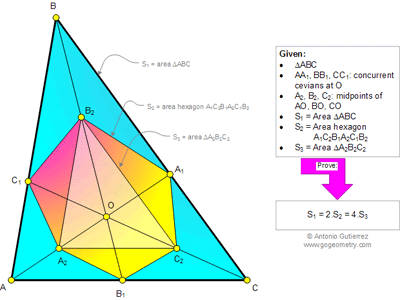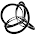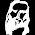## Saturday, February 15, 2014

### Geometry Problem 981. Triangle, Concurrent Cevians, Midpoints, Area, Hexagon

Geometry Problem
Level: Mathematics Education, High School, Honors Geometry, College.

Click the figure below to see the complete problem 981.#### 2 comments:

1.By an affine transformation to an equilateral triangle, Triangle A2B2C2 is also an equiliateral triangle with sides lengths half of A1B1C1.
So S1 = 4*S3

And the hexagon is also a regular ones such that area of triangle A1B2C2 = area of triangle OB2C2, symmetrical for the other 3 triangles.
So S2 = 2*S3

Q.E.D.

2.Let S(ABC) be the area of ABC.

Under the homothetic transformation with center O and scale factor 2,
we have A₂B₂C₂→ABC. Hence, S₁=4S₃.

Now since
B₂C₂ bisects OA₁
A₂C₂ bisects OB₁
A₂B₂ bisects OC₁

Thus
S(OB₂C₂)=S(A₁B₂C₂)
S(OA₂C₂)=S(B₁A₂C₂)
S(OA₂B₂)=S(C₁A₂B₂)

Therefore, S₂=2S₃.

Hence, S₁=2S₂=4S₃.